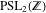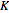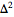# Volume 18

## Stochastic Simulation and Parameter Estimation of the FitzHugh–Nagumo Model »NBCDFPDF

Published October 24, 2016

This article illustrates how Mathematica can be employed to model stochastic processes via stochastic differential equations to compute trajectories and their statistical features. In addition, we discuss parameter estimation of the model via the maximum likelihood method with global optimization. We consider handling modeling error, system noise and measurement error, compare the stochastic and deterministic models and use the likelihood-ratio test to verify the possible improvement provided by stochastic modeling. The Mathematica code is simple and can be easily adapted to similar problems. Read More »

## Inversive Geometry »NBCDFPDF

### Part 2: Concyclic Points, Tangents, the Riemann Sphere, Rings of Four Circles and the Sierpinski Sieve

Published August 10, 2016

This series of articles showcases a variety of applications around the theme of inversion, which constitutes a strategic way of manipulating configurations of circles and lines in the plane. This article includes the Riemann sphere, rings of four tangent circles, and inverting the Sierpinski sieve. Read More »

## Manipulating Subgroups of the Modular Group »NBCDFPDF

Published May 18, 2016

We describe efficient algorithms for working with subgroups of. Operations discussed include join and meet, congruence testing, congruence closure, subgroup testing, cusp enumeration, supergroup lattice, generators and coset enumeration, and constructing a group from a list of generators. Read More »

## The Poisson-Influenced K-Means Algorithm »NBCDFPDF

### A Maximum-Likelihood Procedure for Clusters with a Known Probability Distribution

Published April 25, 2016

We present an implementation of the Poisson-Influenced-Means Algorithm (PIKA), first developed to characterize the output of a superconducting transition edge sensor (TES) in the few-photon-counting regime. The algorithm seeks to group data into several clusters that minimize their distances from their means, as in classical-means clustering, but with the added knowledge that the cluster sizes should follow a Poisson distribution. Read More »

## Exact and Approximate Solutions of the Abel–Volterra Equations »NBCDFPDF

Published February 27, 2016

Picards iteration is used to find the analytical solutions of some AbelVolterra equations. For many equations, the integrals involved in Picards iteration cannot be evaluated. The author approximates the solutions of those equations employing a semi-implicit product midpoint rule.The Aitkenextrapolation is used to accelerate the convergence of both methods. A blow-up phenomena model, a wave propagation, and a superfluity equation are solved to show the practicality of the methods. Programs offered solve the general equations. A user needs only to enter the particular forcing and kernel functions, constants, and a step size for a particular problem. Read More »

## A Computational Strategy for Effective Gene Silencing through siRNAs »NBCDFPDF

### Designing siRNAs for Gene Silencing

Published January 29, 2016

Biological systems possess traits that are a product of coordinated gene expression, heavily influenced by environmental pressures. This is true of both desirable and undesirable traits (i.e. disease). In cases of unwanted traits, it would be tremendously useful to have a means of systematically lowering the expression of genes implicated in producing the undesirable traits. This article describes the implementation of a computational biology algorithm designed to compute small interfering RNA sequences, capable of systematically silencing the expression of specific genes. Read More »